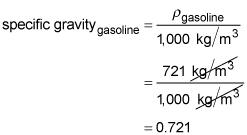##### Physics I: 501 Practice Problems For Dummies (+ Free Online Practice)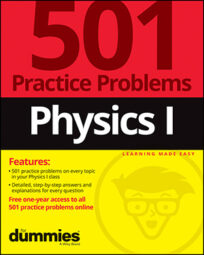Using physics, you can show how mass and volume are related to density. You can also calculate the specific gravity of a material, such as gasoline, if you know its density.

Here are some practice questions that you can try.

## Practice questions

1. You make a cake that has a mass of 300 grams and fits in a cake pan that is 30 by 10 by 6.0 centimeters cubed. What is the density of the cake?

2. A box of cough drops has a mass of 1.0 gram, and its dimensions are 1.0 by 5.0 by 8.5 centimeters cubed. It contains 30 cough drops, each of which has a mass of 2.2 grams. What is the density of the box when it is full of cough drops?

3. The density of gasoline is 721 kilograms per cubic meter. What is its specific gravity?

The following are the answers to the practice questions:

1.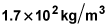Density is mass per unit volume, or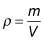In this case, the mass is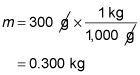The volume is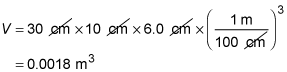Insert these values into the equation for density to find the density of the cake: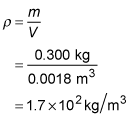2.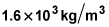Density is mass per unit volume, orThe total mass of the box plus cough drops is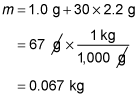The volume of the box is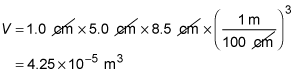Insert these values into the equation for density to find the density of the box: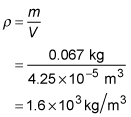3. 0.721

Specific gravity is the density of a material divided by the density of water at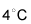which is 1,000 kilograms per cubic meter. The equation for specific gravity is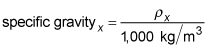The subscript x refers to the material in question. You know that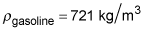Insert this value into the equation for specific gravity to find